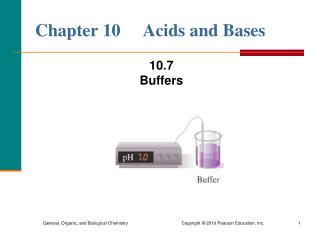DownloadDownload PresentationChapter 10 Acids and Bases

# Chapter 10 Acids and Bases

Télécharger la présentation## Chapter 10 Acids and Bases

- - - - - - - - - - - - - - - - - - - - - - - - - - - E N D - - - - - - - - - - - - - - - - - - - - - - - - - - -
##### Presentation Transcript

1. Chapter 10 Acids and Bases 10.7Buffers

2. Buffers Buffers • resist changes in pH from the addition of acid orbase • in the body absorb H3O+ or OH from foods and cellular processes to maintain pH • are important in the proper functioning of cells and blood • in blood maintain a pH close to 7.4; a change in the pH of the blood affects the uptake of oxygen and cellular processes

3. Buffers (continued) When an acid or base is added • to water, the pH changes drastically • to a buffer solution, the pH does not change very much; pH is maintained

4. Components of a Buffer The components of a buffer solution • are acid–base conjugate pairs • can be a weak acid and a salt of its conjugate base • typically have equal concentrations of the weak acid and its salt • can also be a weak base and a salt of its conjugate acid

5. Learning Check Does each of the following combinations produce a buffer solution or not? Explain. A. HCl and KCl B. H2CO3 and NaHCO3 C. H3PO4 and NaCl D. HC2H3O2 and KC2H3O2

6. Solution Does each of the following combinations produce a buffer solution or not? Explain. A. HCl + KCl No; HCl is a strong acid. B. H2CO3 + NaHCO3 Yes; this is a weak acid and its salt C. H3PO4 + NaCl No; NaCl does not contain a conjugate base of H3PO4. D. HC2H3O2 and KC2H3O2 Yes; this is a weak acid and its salt

7. Buffer Action An acetic acid/acetate buffer contains the weak acid acetic acid (HC2H3O2) and the salt of its conjugate base sodium acetate (NaC2H3O2). • Acid dissociation occurs: HC2H3O2(aq) + H2O(l) C2H3O2(aq) + H3O+(aq) • The salt provides a higher concentration of the conjugate base C2H3O2 than provided by the dissociation of the weak acid by itself. NaC2H3O2(aq) Na+(aq) + C2H3O2(aq)

8. Function of the Weak Acid in a Buffer • The function of the weak acid in a buffer is to neutralize added base. • The acetate ion produced by the neutralization becomes part of the available acetate. HC2H3O2(aq) + OH−(aq) C2H3O2(aq) + H2O(l) acetic acid base acetate ion water

9. Function of the Conjugate Base • The function of the acetate ion C2H3O2 is to neutralize added H3O+. • The acetic acid produced by the neutralization contributes to the available weak acid. C2H3O2(aq) + H3O+(aq) HC2H3O2(aq)+ H2O(l) acetate ion acid acetic acid water

10. Summary of Buffer Action Buffer action occurs because • the weak acid in a buffer neutralizes base • the conjugate base in the buffer neutralizes acid • the pH of the solution is maintained

11. pH of a Buffer The [H3O+] in the Ka expression is used to determine the pH of a buffer. Weak acid + H2O H3O+ + conjugate base Ka = [H3O+][conjugate base] [weak acid] [H3O+] = Ka x [weak acid] [conjugate base] pH = log [H3O+]

12. Guide to Calculating pH of a Buffer

13. Example of Calculating Buffer pH The weak acid H2PO4 in a blood buffer H2PO4/HPO42 has a Ka = 6.2 x 108. What is the pH of the buffer if [H2PO4] = 0.20 M and [HPO42] = 0.20 M? STEP 1 Write the Ka expression for: H2PO4(aq) + H2O(l) HPO42(aq) + H3O+(aq) Ka = [HPO42][H3O+] [H2PO4] STEP 2 Rearrange the Ka for [H3O+]: [H3O+] = Ka x [H2PO4] [HPO42]

14. Example of Calculating Buffer pH (continued) STEP 3Substitute [HA] and [A]: [H3O+] = 6.2 x 108 x [0.20 M] = 6.2 x 108 [0.20 M] STEP 4Use [H3O+] to calculate pH: pH =  log [6.2 x 10 8] = 7.21

15. Learning Check What is the pH of a H2CO3 buffer that is 0.20 M H2CO3 and 0.10 M HCO3? Ka(H2CO3) = 4.3 x 107 1) 6.67 2) 6.37 3) 6.07

16. Solution What is the pH of a H2CO3 buffer that is 0.20 M H2CO3 and 0.10 M HCO3? Ka(H2CO3) = 4.3 x 107 STEP 1 Write the Ka expression for: H2CO3(aq) + H2O(l) HCO3(aq) + H3O+(aq) Ka = [HCO3][H3O+] [H2CO3] STEP 2 Rearrange the Ka for [H3O+]: [H3O+] = Ka x [H2CO3] [HCO3]

17. Solution (continued) STEP 3Substitute [HA] and [A]: [H3O+] = 4.3 x 107 x [0.20 M] = 8.6 x 107 M [0.10 M] STEP 4Use [H3O+] to calculate pH: pH =  log [8.6 x 107] = 6.07